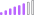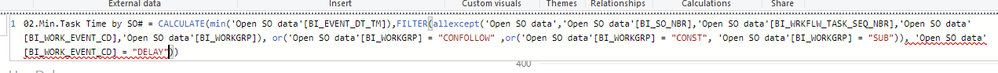cancel
Showing results for
Did you mean:

Fabric is Generally Available. Browse Fabric Presentations. Work towards your Fabric certification with the Cloud Skills Challenge.Post Patron

## Help with some Syntax

If someone can help me figure out how to fix my syntax I'd appreciate it. In the picture you can see that powerbi doesn't like my last section where I have the DELAY condition added. If I add it before the "OR" section of code that the error switches to the OR statement.

02.Min.Task Time by SO# = CALCULATE(min('Open SO data'[BI_EVENT_DT_TM]),FILTER(allexcept('Open SO data','Open SO data'[BI_SO_NBR],'Open SO data'[BI_WRKFLW_TASK_SEQ_NBR],'Open SO data'[BI_WORK_EVENT_CD],'Open SO data'[BI_WORKGRP]), or('Open SO data'[BI_WORKGRP] = "CONFOLLOW" ,or('Open SO data'[BI_WORKGRP] = "CONST", 'Open SO data'[BI_WORKGRP] = "SUB")), 'Open SO data'[BI_WORK_EVENT_CD] = "DELAY"))1 ACCEPTED SOLUTIONSuper User

Hi @GunnerJ

You seem to be using FILTER with three arguments. It takes only two. Try this, where I'm assuming you want and AND

```02.Min.Task Time by SO# =
CALCULATE (
MIN ( 'Open SO data'[BI_EVENT_DT_TM] ),
FILTER (
ALLEXCEPT (
'Open SO data',
'Open SO data'[BI_SO_NBR],
'Open SO data'[BI_WORK_EVENT_CD],
'Open SO data'[BI_WORKGRP]
),
OR (
'Open SO data'[BI_WORKGRP] = "CONFOLLOW",
OR ( 'Open SO data'[BI_WORKGRP] = "CONST", 'Open SO data'[BI_WORKGRP] = "SUB" )
)
&& 'Open SO data'[BI_WORK_EVENT_CD] = "DELAY"
)
)
```

or this (I don't know what you are trying to do)

```02.Min.Task Time by SO# =
CALCULATE (
MIN ( 'Open SO data'[BI_EVENT_DT_TM] ),
FILTER (
ALLEXCEPT (
'Open SO data',
'Open SO data'[BI_SO_NBR],
'Open SO data'[BI_WORK_EVENT_CD],
'Open SO data'[BI_WORKGRP]
),
OR (
'Open SO data'[BI_WORKGRP] = "CONFOLLOW",
OR ( 'Open SO data'[BI_WORKGRP] = "CONST", 'Open SO data'[BI_WORKGRP] = "SUB" )
)
),
'Open SO data'[BI_WORK_EVENT_CD] = "DELAY"
)
```
2 REPLIES 2Super User

Hi @GunnerJ

You seem to be using FILTER with three arguments. It takes only two. Try this, where I'm assuming you want and AND

```02.Min.Task Time by SO# =
CALCULATE (
MIN ( 'Open SO data'[BI_EVENT_DT_TM] ),
FILTER (
ALLEXCEPT (
'Open SO data',
'Open SO data'[BI_SO_NBR],
'Open SO data'[BI_WORK_EVENT_CD],
'Open SO data'[BI_WORKGRP]
),
OR (
'Open SO data'[BI_WORKGRP] = "CONFOLLOW",
OR ( 'Open SO data'[BI_WORKGRP] = "CONST", 'Open SO data'[BI_WORKGRP] = "SUB" )
)
&& 'Open SO data'[BI_WORK_EVENT_CD] = "DELAY"
)
)
```

or this (I don't know what you are trying to do)

```02.Min.Task Time by SO# =
CALCULATE (
MIN ( 'Open SO data'[BI_EVENT_DT_TM] ),
FILTER (
ALLEXCEPT (
'Open SO data',
'Open SO data'[BI_SO_NBR],
'Open SO data'[BI_WORK_EVENT_CD],
'Open SO data'[BI_WORKGRP]
),
OR (
'Open SO data'[BI_WORKGRP] = "CONFOLLOW",
OR ( 'Open SO data'[BI_WORKGRP] = "CONST", 'Open SO data'[BI_WORKGRP] = "SUB" )
)
),
'Open SO data'[BI_WORK_EVENT_CD] = "DELAY"
)
```Post Patron

@AlB I see my mistake. Thank you for the quick reply!Announcements#### Power BI Monthly Update - November 2023

Check out the November 2023 Power BI update to learn about new features.#### Fabric Community News unified experience

Read the latest Fabric Community announcements, including updates on Power BI, Synapse, Data Factory and Data Activator.#### Exclusive opportunity for Women!

Join us for a free, hands-on Microsoft workshop led by women trainers for women where you will learn how to build a Dashboard in a Day!#### The largest Power BI and Fabric virtual conference

130+ sessions, 130+ speakers, Product managers, MVPs, and experts. All about Power BI and Fabric. Attend online or watch the recordings.Top Solution Authors
Top Kudoed Authors
Users online (5,089)